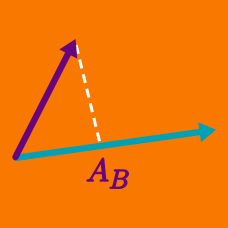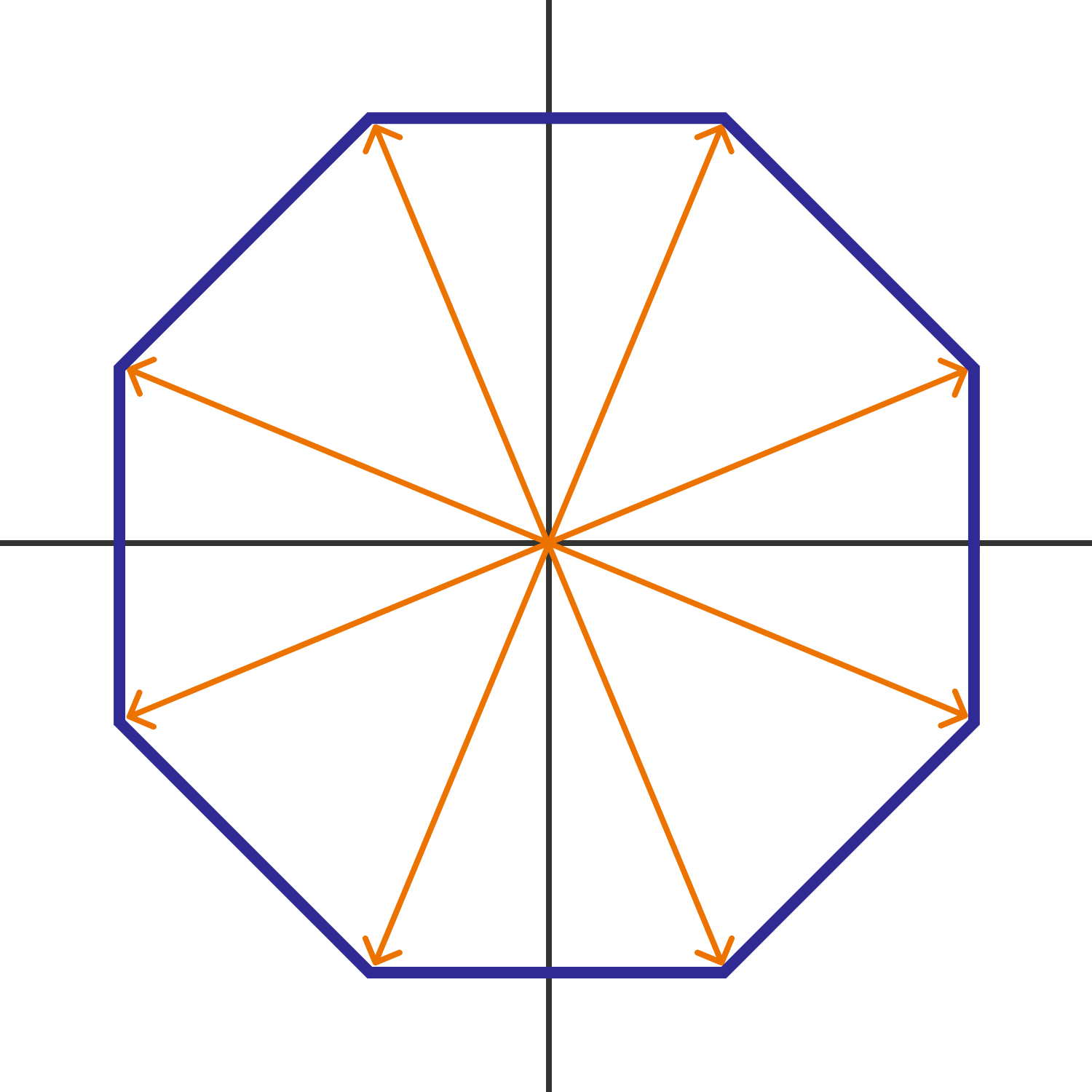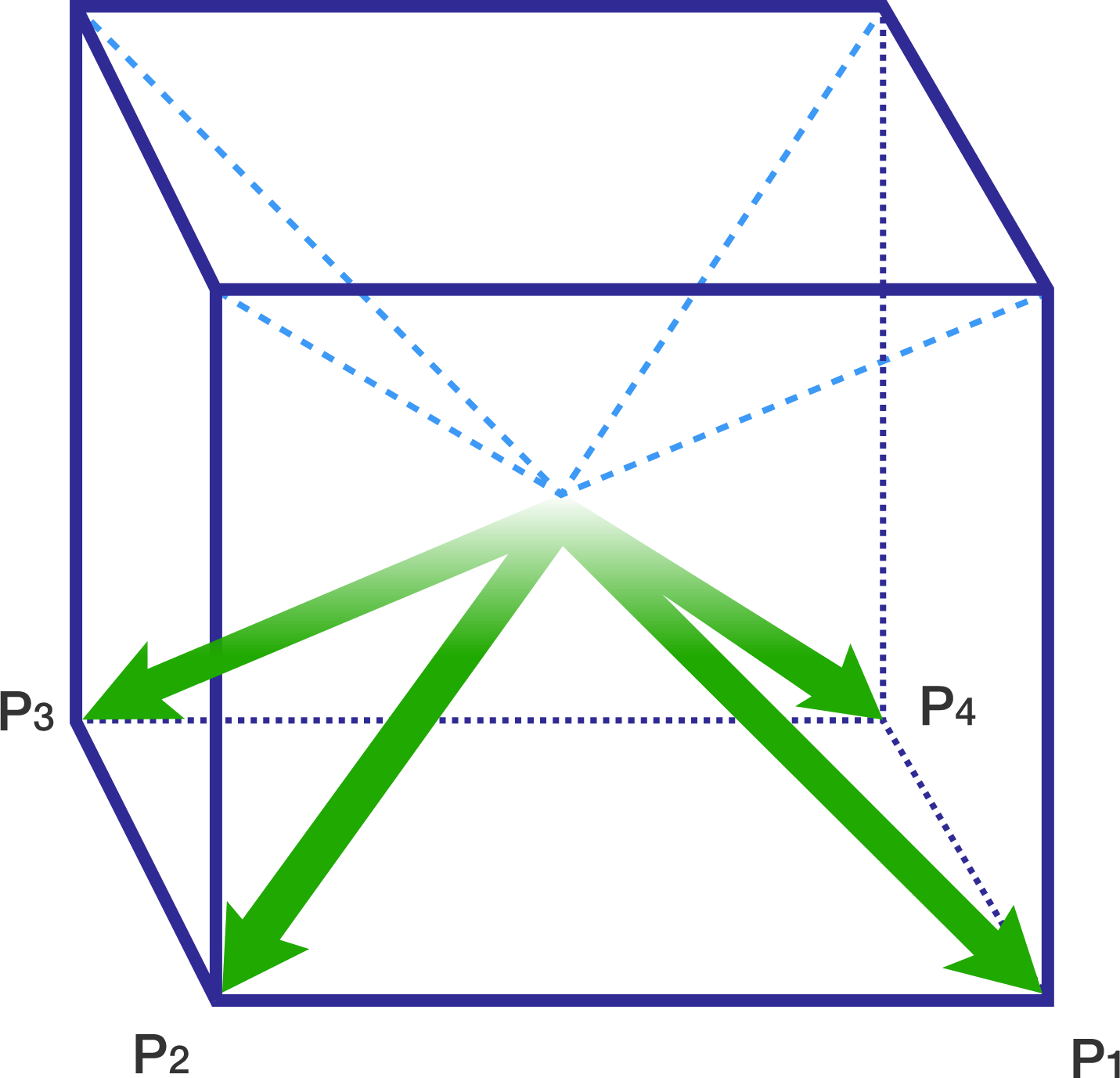Geometry

# Dot Product of Vectors: Level 3 Challenges

Three points $$A, B,$$ and $$C$$ in three-dimensional Euclidean space have their respective coordinates $$(-6, 2, –4), (–1, 1, –2),$$ and $$(-2, 2, 1).$$ What is the measure of $$\angle ABC?$$Consider the regular octagon centered at the origin as shown above. Eight unit vectors are drawn from the center of the octagon to each of its vertices. For each pair of distinct unit vectors, the dot product is computed. What is the sum of all of these dot products?If a line makes angles $$\alpha$$ , $$\beta$$ , $$\gamma$$ and $$\delta$$ with the four body diagonals of a cube and the value of
$\cos^2(\alpha) + \cos^2(\beta) + \cos^2(\gamma) + \cos^2(\delta)$ can be expressed as $$\frac{p}{q}$$, where $$p$$ and $$q$$ are coprime integers, find the value of $$p + q$$.

Clarification: Body diagonals of a cube are the diagonals which do not lie along any face of the cube.

Let $$a_1, \ldots, a_{16}$$ be the list of $$2^4 = 16$$ distinct vectors which have 4 coordinates, whose values are either 0 or 1. What is the maximum possible value of $$a_1 \cdot a_2 + a_3 \cdot a_4 + \cdots + a_{15} \cdot a_{16}$$?

Details and assumptions

$$u \cdot v$$ represents the dot product of vectors.

Examples of vectors which have 4 coordinates and whose entries are either 0 or 1 are: $$(0, 0, 0, 0), (1, 1, 1, 1), (0, 1, 0, 1), (1, 0, 0, 0)$$.

The list is a set of all the 16 distinct vectors which satisfy the condition.

Given two vectors $$\vec{u}$$ and $$\vec{v}$$ such that $$\|\vec{u}\| = 5$$ and $$\|\vec{v}\| = 8$$, what is the positive difference between the largest and smallest possible values of $$\vec{u}\cdot\vec{v}?$$

×

Problem Loading...

Note Loading...

Set Loading...